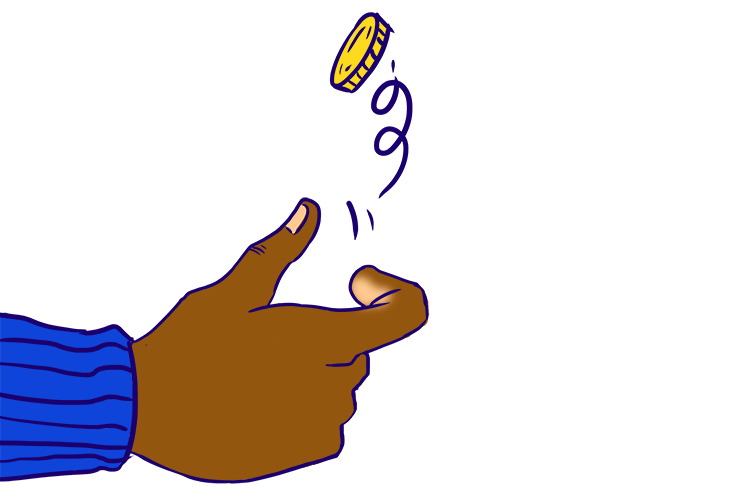# The most famous probability examples

Example 1

Throwing a dice:

A dice has six sides – 1,2,3,4,5 and 6 dots.So\ Probability = (RIGHT)/(ALL)=(The\ n\umber\ of\ ways\ of\ ach\ievi\ng\ sucess)/(The\ n\umber\ of\ possibl\e\ outcomes

Probability=1/6

Example 2

Tossing a coin – Heads or tailsSo\ Probability = (RIGHT)/(ALL)=(The\ n\umber\ of\ ways\ of\ ach\ievi\ng\ sucess)/(The\ n\umber\ of\ possibl\e\ outcomes

Probability=1/2